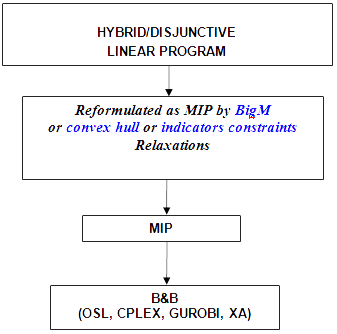# Linear Solvers

The following figure shows how the solution for linear hybrid/disjunctive models is driven:The disjunctions defined in the model are transformed into mixed integer formulations by using one of the relaxations proposed: BigM or convex hull or indicators constraints. The complete set of disjunctions can be transformed by one of those relaxations, or you can choose a different one for each disjunction in the model. Then, the problem is converted into a Mixed Integer Program (MIP) which is later solved by a Branch and Bound algorithm. References about the relaxations can be found in Balas(1979), Vecchietti and Grossmann(2002).

The default relaxation is the convex hull. You can change it by introducing in the LogMIP section the following sentence:

DEFAULT Big-M

By means of this sentence disjunctions are relaxed using the Big-M relaxation. Since LogMIP 2.0 belongs to the EMP environment, to solve the problem you must write in the GAMS input file the following two sentences:

OPTION EMP=LOGMIP;
SOLVE modelname USING EMP [MINIMIZING | MAXIMIZING] variablename